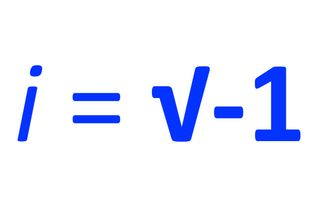# What Are Imaginary Numbers?An imaginary number is a number that, when squared, has a negative result. Essentially, an imaginary number is the square root of a negative number and does not have a tangible value. While it is not a real number — that is, it cannot be quantified on the number line — imaginary numbers are "real" in the sense that they exist and are used in math.

Imaginary numbers, also called complex numbers, are used in real-life applications, such as electricity, as well as quadratic equations. In quadratic planes, imaginary numbers show up in equations that don’t touch the x axis. Imaginary numbers become particularly useful in advanced calculus.

Usually denoted by the symbol i, imaginary numbers are denoted by the symbol j in electronics (because i already denotes "current"). Imaginary numbers are particularly applicable in electricity, specifically alternating current (AC) electronics. AC electricity changes between positive and negative in a sine wave. Combining AC currents can be very difficult because they may not match properly on the waves. Using imaginary currents and real numbers helps those working with AC electricity do the calculations and avoid electrocution.

Imaginary numbers can also be applied to signal processing, which is useful in cellular technology and wireless technologies, as well as radar and even biology (brain waves). Essentially, if what is being measured relies on a sine or cosine wave, the imaginary number is used.

## Imaginary numbers chart

There is also an interesting property of i. When you multiply it, it cycles through four different values. For example, i x i = -1. Then, -1 x i = -i. -i x i = 1. Then 1 x i = i, coming full circle. This makes exponents of i easy to figure out. If:

This cycle will continue through the exponents, also known as the imaginary numbers chart. Knowledge of the exponential qualities of imaginary numbers is useful in the multiplication and division of imaginary numbers. After grouping the coefficients and the imaginary terms, the rules of exponents can be applied to i while the real numbers are multiplied as normal. The same is done with division. By applying the usual multiplication and division rules, imaginary numbers can be simplified as you would with variables and coefficients.

Imaginary numbers have also made an appearance in pop culture. In Dan Brown’s "The Da Vinci Code," protagonist Robert Langdon refers to Sophie Neveu’s belief in the imaginary number. Isaac Asimov has also used imaginary numbers in his short stories, like “The Imaginary,” where imaginary numbers and equations describe the behavior of a species of squid.